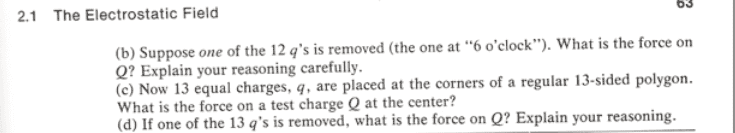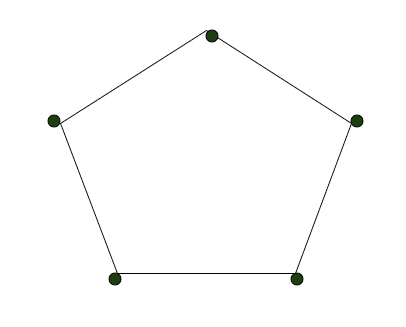# Simple Electrostatics Problem (Conceptual)

In summary, the conversation is about finding the center of a regular polygon, specifically a 13-sided polygon, in order to determine the net force at the center due to the charges at the vertices. The definition of the center is discussed, and it is determined that the center can be located by drawing lines from each vertex to the midpoint of the opposite side, or by drawing perpendiculars from the center of each side. The concept of symmetry is also brought up, and it is explained that in a regular polygon, the forces from the charges at the vertices will cancel out at the center due to the arrangement of the charges.

Part CThe answer is zero. But I am not sure what I am missing. I am using a Pentagon to do the analysis (I don't feel like drawing a 13-sided object).

It seems like I need to know a little more about the geometry of a Pentagon. How is the center of an odd-number-of-sides polygon defined?

I am assuming that in is not equidistant from ALL of the vertices? Otherwise i don't see how it could be zero.

Am I making this more difficult than it is? Am I just supposed to look up the formula(s) for the center of a 13-sided polygon?Before the q was removed, the force at the center is zero due to symmetry. This means that the force due to the q is equal and opposite to the net force due to the rest of the charges.

dx said:
Before the q was removed, the force at the center is zero due to symmetry. This means that the force due to the q is equal and opposite to the net force due to the rest of the charges.

But if it is 13-sided, how is it symmetrical? There would be 1 unpaired charge.

It's not about pairing, its the way they are arranged. Think about an equilateral triangle with 3 charges at the tips. The force at the center will be zero.

But if it is 13-sided, how is it symmetrical? There would be 1 unpaired charge.
There are no pairs. There would be pairs if you had two charges directly across from each other, then you could call them a "pair" and their forces would cancel out. And that does indeed happen in a polygon with an even number of sides. But in a regular 13-sided polygon, the "thing" across from a charge is just the empty space between two charges. (i.e. a side of the polygon) So you can't talk about pairs of charges.

It turns out that when you add up the forces from the charges at the corners of a regular 13-sided polygon (or any regular polygon), at the center of the polygon, the forces cancel out. You can see this by figuring out what the forces actually are (as vectors) and by adding them up, but there's an easier way: by symmetry. Think about this: if there were a force on a charge at the center, which way would it point?

Okay. That make sense. I guess that my confusion lies in the definition of the center. When we speak of the "centers" of polygons, we are speaking of the centers of mass, right?

The vertices of a regular polygon can always be made to fit on the the circumference of a circle, so you can think of the center as the center of the circle.

dx said:
The vertices of a regular polygon can always be made to fit on the the circumference of a circle, so you can think of the center as the center of the circle.
Yeah, that's it. A polygon is just a mathematical idea, not an actual physical object, so it has no mass (and therefore no center of mass).

I am also confused because you keep saying "symmetry." I don't see what is symmetrical here.diazona said:
Yeah, that's it. A polygon is just a mathematical idea, not an actual physical object, so it has no mass (and therefore no center of mass).

I am using COM as a tool here. I know that shapes don't have massWell, what exactly do you think is the definition of "symmetry"?

Draw lines from the center to each of the vertices. The angles between adjacent lines will be the same.

dx said:
Draw lines from the center to each of the vertices. The angles between adjacent lines will be the same.

I was actually just trying to do this when I re-encountered the question about the center again.

If I want to locate the center of a pentagon, without using a circle, how would I find it?

Would I draw a line from each vertex to the midpoint of the opposite side? And their intersection would be the center?

Draw a perpendicular from the center of each of the sides. They will intersect at the center.

I was actually just trying to do this when I re-encountered the question about the center again.

If I want to locate the center of a pentagon, without using a circle, how would I find it?

Would I draw a line from each vertex to the midpoint of the opposite side? And their intersection would be the center?
If it's a regular pentagon, yes, that is one way that would work. (If it's not a regular pentagon, there are multiple ways to define the "center" and they give different points, so it's kind of a meaningless question... but let's not worry about that ;-)

diazona said:
If it's a regular pentagon, yes, that is one way that would work. (If it's not a regular pentagon, there are multiple ways to define the "center" and they give different points, so it's kind of a meaningless question... but let's not worry about that ;-)

I am not trying to nit-pick here, but how can the question be meaningless if what I am doing is finding the net force at the center ? The force depends on how we define the center, does it not? If I am given that charges lie at each of the vertices, and a test charge at the center, than the most meaningful question that I can ask is: how are the distances from each vertex to the center defined ;-)

Now since the problem statement gives that it is a regular polygon, I can find the center as stated above.

Now what I said
draw a line from each vertex to the midpoint of the opposite side and their intersection would be the center

and what dx said
Draw a perpendicular from the center of each of the sides. They will intersect at the center.

do not appear to be the same thing. So which way (if either) is correct?